How to Calculate the Compound Annual Growth Rate "CAGR" in Microsoft Excel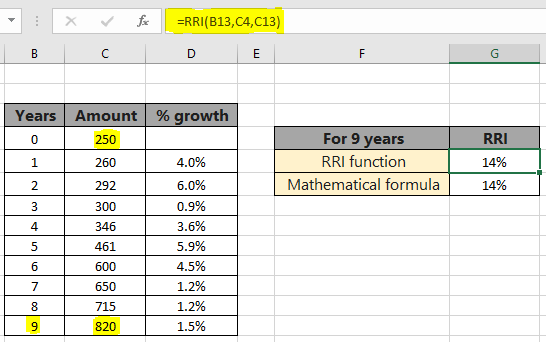In this article, we will learn How to Calculate the Compound Annual Growth Rate "CAGR" in Microsoft Excel.

What is CAGR ?

Compound annual growth rate (CAGR) is a business and investment term that provides a constant rate of return over the time. It can be thought of as the growth rate that gets you from the initial investment value to the ending investment value if you assume that the investment has been compounding over the time.

RRI is the equivalent interest rate for growth of an investment. Generally it is used to calculate the Compound Annual Growth Rate (CAGR). It returns the interest rate for the given period of time having future and present value of investment. The mathematical formula to calculate CAGR or RRI value is shown below.

RRI or CAGR = [(FV/PV) ^ (1/nper)] - 1

Here nper is the time period over which the rri value is calculated. Let's understand how to calculate CAGR in excel.

RRI function in Excel

RRI function in excel returns the equivalent interest rate over a given period of time. This interest rate is calculated for the difference between present value and future value of investment. Let's see the below function syntax to understand.

RRI Function syntax:

 =RRI(nper, pv, fv)

nper : period of time in years

pv : present value of investment

fv : future value of investment

Example :

All of these might be confusing to understand. Let's understand how to use the function using an example. Here we are given a list of amounts over a period of 9 years. We need to calculate the Annual Growth Rate for the following data. First we will calculate the annual growth percentage for the first year.

Use the formula:

 =RRI (1, C4, C5)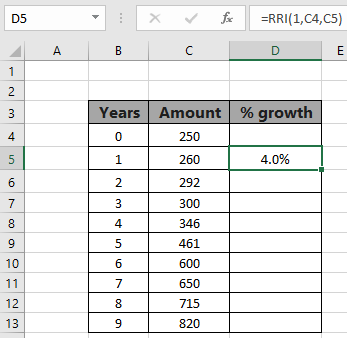As you can see there is 4 percent growth in Sales for the first year. Now extend this formula to the remaining cells using the Ctrl + D or dragging down from the right bottom of the formula acquired cell.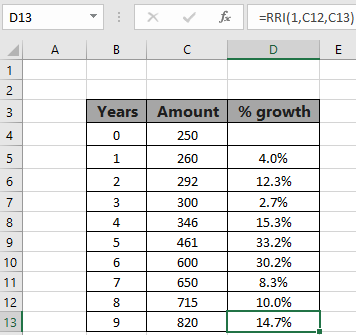This array indicates the annual growth in each year comparing value in the start of period (pv) with the value at the end of period (fv). This function allows you to choose the time interval between two values. Now we will calculate the equivalent interest for the whole 9 years.

Use the formula:

 =RRI (9, C4, C13)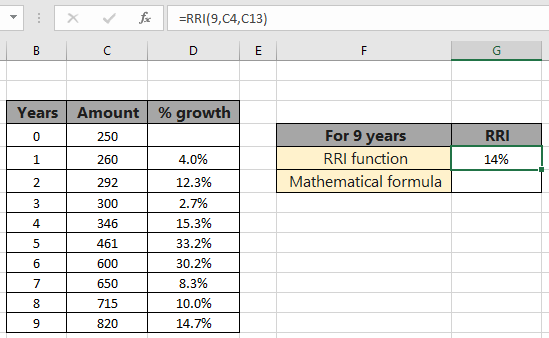The equivalent compound annual growth rate comes out to be 14%. The formula might not return the value in percentage, it might be showing 0.141 (in decimals). Change the format of the cell from Number to Percentage.

Calculate RRI using CAGR formula in excel

Calculate the same result using the mathematical formula stated formula. CAGR = [(FV/PV) ^ (1/nper)] - 1. This ^ character indicates the power of the base value.

Use the formula:

 =(C13/C4)^(1/9)-1

C13 : future value

C4 : present value

9 : period of investment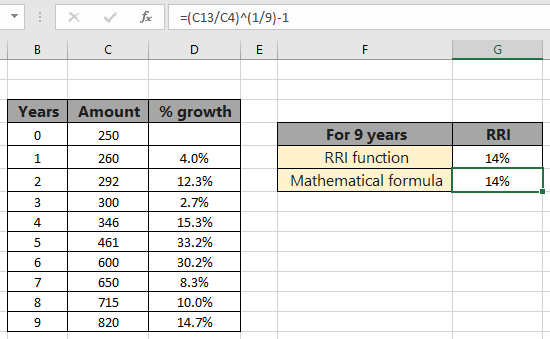The formula returns 14%, the same value returned by the RRI function. Functions are easy to use and there are less chances of error, than using the mathematical formula.

Here are some observational notes using the RRI function shown below.
Notes :

1. The function returns the equivalent interest rate in decimal (0.12) or percent (12%) value. The both are considered same in Excel
2. If any argument of the function has negative value, the function returns #NUM! Error.
3. If any argument of the function has non numeric value, the function returns #VALUE! error.

Hope this article about How to Calculate the Compound Annual Growth Rate "CAGR" in Microsoft Excel is explanatory. Find more articles on calculating values and related Excel formulas here. If you liked our blogs, share it with your friends on Facebook. And also you can follow us on Twitter and Facebook. We would love to hear from you, do let us know how we can improve, complement or innovate our work and make it better for you. Write to us at info@exceltip.com.

Related Articles

How to use the MIRR function in excel : returns the Modified interest rate of return for the financial data having Investment, finance rate & reinvestment_rate using the MIRR function in Excel.

How to use the XIRR function in excel : returns the Interest rate of return for irregular interval using the XIRR function in Excel

Excel PV vs FV function : find Present Value using PV function and future value using FV function in Excel.

How to use the RECEIVED function in excel : calculates the amount which is received at maturity for a bond with an initial investment (security) and a discount rate, there are no periodic interest payments using the RECEIVED function in excel.

How to use the NPER function in excel : NPER function to calculate periods on payments in Excel.

How to use the PRICE function in excel : returns the price per \$100 face value of a security that pays periodic interest using the PRICE function in Excel.

Popular Articles :

How to use the IF Function in Excel : The IF statement in Excel checks the condition and returns a specific value if the condition is TRUE or returns another specific value if FALSE.

How to use the VLOOKUP Function in Excel : This is one of the most used and popular functions of excel that is used to lookup value from different ranges and sheets.

How to Use SUMIF Function in Excel : This is another dashboard essential function. This helps you sum up values on specific conditions.

How to use the COUNTIF Function in Excel : Count values with conditions using this amazing function. You don't need to filter your data to count specific values. Countif function is essential to prepare your dashboard.

Terms and Conditions of use

The applications/code on this site are distributed as is and without warranties or liability. In no event shall the owner of the copyrights, or the authors of the applications/code be liable for any loss of profit, any problems or any damage resulting from the use or evaluation of the applications/code.